Motion in Space

Copyright © 2001 by James F. Hurley, University of Connecticut Department of Mathematics, Unit 3009, Storrs CT 06269-3009. All rights reserved.

The interactive version of this worksheet is in the Math 210 folder in the Workspace volume of MSB 203.

This worksheet, a continuation of
Space Curves , focuses on motion of a particle along a curve

r ( t ) = x ( t ) i + y ( t ) j + z ( t ) k = < x ( t ), y ( t ), z ( t ) >.

in space. To begin, return to the first curve considered in the previous worksheet, namely the circular helix

r ( t ) = <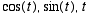>.

It is easy to calculate its veclocity and acceleration vectors, namely,

v ( t ) = <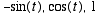> and a ( t ) = <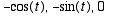>.

Since the speed of a particle moving along the curve is ||
v ( t ) || =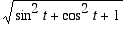=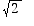, the unit tangent vector is

T ( t ) =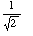<>.

To find the unit normal vector N ( t ), first differentiate T ( t ), and then divide by its length, which for the circular helix is the constant.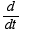[ T ( t )] =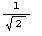<>, so N ( t ) =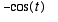i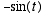j .

The next routine plots the helix, along with its unit tangent and normal vectors at the point where
t =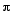/2. It draws the unit tangent vector by plotting the segment of the tangent line

r = < 0, 1,/2 > + t <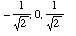>

at the point
P (0, 1,/2) in the direction of the unit tangent vector T = <> for t ranging from 0 to 1. (At t = 0, we are at P and at t = 1 we are at the end of the T (/2) drawn from initial point P .) It uses the same approach for N (/2): starting from P it plots the normal line

r = < 0, 1,/2 > + t <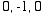>.

for
t between 0 and 1 (where we reach the end of the unit normal vector).

> with (plots):
curve := spacecurve( [cos(t), sin(t), t], t = 0..2*Pi, color = black, axes=boxed, numpoints = 150, labels = ["x", "y", "z"] ):
utan := spacecurve( [-t/sqrt(2), 1, Pi/2 + t/sqrt(2)], t = 0..1, color = blue, axes = boxed, numpoints = 150 ):
unorm := spacecurve( [0, 1 - t, Pi/2], t = 0..1, color = green, axes = boxed, numpoints = 150 ):
xaxis := spacecurve( [t, 0, 0, t = -1..1, color = magenta] ):
yaxis := spacecurve( [0, t, 0, t = -1..1, color = magenta] ):
zaxis := spacecurve( [0, 0, t, t = -1..6, color = magenta] ):
labx := textplot3d([1.2, 0, -.2, `x`], color = magenta):
laby := textplot3d([0,1.2, -.2, `y`], color = magenta):
labz := textplot3d([0, 0, 6.5, `z`], color = magenta):
display(curve, utan, unorm, xaxis, yaxis, zaxis, labx, laby, labz);

```Warning, the name changecoords has been redefined
```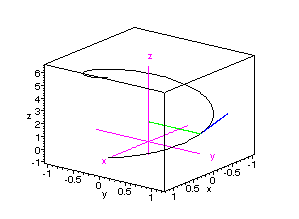The binormal vector is defined to be
B ( t ) = T ( t ) ´ N ( t ). For the helix, this gives

B ( t ) =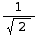<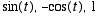>

=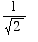<1, 0, 1> for t =/2.

The next routine uses the same technique that plotted
T and N in the preceding plot to add a plot of this binormal vector at t =/2.

> with (plots):
curve := spacecurve( [cos(t), sin(t), t], t = 0..Pi, color = black, axes=boxed, numpoints = 150, labels = ["x", "y", "z"] ):
utan := spacecurve( [-t/sqrt(2), 1, Pi/2 + t/sqrt(2)], t = 0..1, color = blue, axes = boxed, numpoints = 150 ):
unorm := spacecurve( [0, 1 - t, Pi/2], t = 0..1, color = green, axes = boxed, numpoints = 150 ):
binorm := spacecurve( [t/sqrt(2), 1, Pi/2 + t/sqrt(2)],t = 0..1, color = brown, axes = boxed, numpoints = 150 ):
xaxis := spacecurve( [t, 0, 0, t = -1..1, color = magenta] ):
yaxis := spacecurve( [0, t, 0, t = -1..1, color = magenta] ):
zaxis := spacecurve( [0, 0, t, t = -1..4, color = magenta] ):
labx := textplot3d([1.2, 0, -.2, `x`], color = magenta):
laby := textplot3d([0,1.2, -.2, `y`], color = magenta):
labz := textplot3d([0, 0, 4.5, `z`], color = magenta):
display(curve, utan, unorm, binorm, xaxis, yaxis, zaxis, labx, laby, labz, scaling = constrained);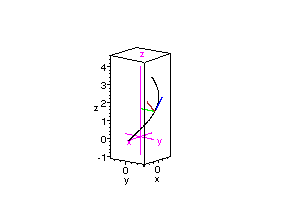Note. To display the right angles between the unit normal and unit tangent vectors properly, the command scaling = constrained was added to the display command. It does not appear in the interactive version of this worksheet.

Example. Exercise 8, Section 14.4 of Stewart's Calculus, 4th Edition, asks for a plot of the parametric curve and corresponding velocity and acceleration vectors with vector representation r ( t ) = <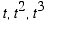> for t = 1.
Solution
. Since v ( t ) = <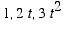> = <1, 2, 3> at t = 1 and a ( t ) = < 0, 2,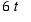> = < 0, 2, 6 > there, the following routine provides the required image. As above, it plots the parts of the tangent line and "acceleration line" through the point P (1, 1, 1) on the curve corresponding to t = 1 in the respective directions of the velocity and acceleration vectors.

> with (plots):
curve := spacecurve( [t, t^2, t^3], t = 0..2, color = black, axes=boxed, numpoints = 150, labels = ["x", "y", "z"] ):
veloc := spacecurve( [1 + t, 1 + 2*t, 1 + 3*t], t = 0..1, color = blue, axes = boxed, numpoints = 150 ):
accel := spacecurve( [1, 1 + 2*t, 1 + 6*t], t = 0..1, color = green, axes = boxed, numpoints = 150 ):
xaxis := spacecurve( [t, 0, 0, t = -1..1, color = magenta] ):
yaxis := spacecurve( [0, t, 0, t = -1..1, color = magenta] ):
zaxis := spacecurve( [0, 0, t, t = -1..6, color = magenta] ):
labx := textplot3d([1.2, 0, -.2, `x`], color = magenta):
laby := textplot3d([0,1.2, -.2, `y`], color = magenta):
labz := textplot3d([0, 0, 6.5, `z`], color = magenta):
display(curve, veloc, accel, xaxis, yaxis, zaxis, labx, laby, labz);

It is easy to decompose the accleration vector a (1) into its tangential and normal components of the acceleration at the point P (1, 1, 1) corresponding to t = 1. From the calculations above, for instance,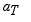(1) = [ (a á v)/(v á v)] v = [22/14] < 1, 2, 3 > = < 11/7, 22/7, 33/7 >.

Then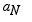(1) =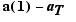(1) =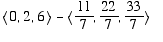=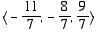.

From this, it is easy to calculate

N (1) = [1/||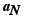(1) ||](1) =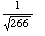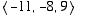.

The next routine plots the accleration and its tangential and normal components at t = 1.

> with (plots):
curve := spacecurve( [t, t^2, t^3], t = 0..2, color = black, axes=boxed, numpoints = 150, labels = ["x", "y", "z"] ):
accel := spacecurve( [1, 1 + 2*t, 1 + 6*t], t = 0..1, color = green, axes = boxed, numpoints = 150 ):
asubt := spacecurve( [1 + 11*t/7, 1 + 22*t/7, 1 + 33*t/7], t = 0..1, color = blue, axes = boxed):
asubn := spacecurve( [1 - 11*t/7, 1 - 8*t/7, 1 + 9*t/7], t = 0..1, color = blue, axes = boxed ):
parall1 := spacecurve( [18/7 - 11*t/7, 29/7- 8*t/7, 40/7 + 9*t/7], t = 0..1, color = cyan, axes = boxed ):
parall2 := spacecurve( [-4/7 + 11*t/7, -1/7 + 22*t/7, 16/7 + 33*t/7], t = 0..1, color = cyan, axes = boxed ):
xaxis := spacecurve( [t, 0, 0, t = -1..1, color = magenta] ):
yaxis := spacecurve( [0, t, 0, t = -1..1, color = magenta] ):
zaxis := spacecurve( [0, 0, t, t = -1..6, color = magenta] ):
labx := textplot3d([1.2, 0, -.2, `x`], color = magenta):
laby := textplot3d([0,1.2, -.2, `y`], color = magenta):
labz := textplot3d([0, 0, 6.5, `z`], color = magenta):
display(curve, accel, asubt, asubn, parall1, parall2, xaxis, yaxis, zaxis, labx, laby, labz);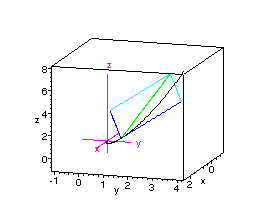>

Finally, note that this decomposition of
a (1) provides a quick way to calculate the curvature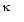(1) at t = 1 without the necessity for any ugly differentiating or computation of a cross product. Since the normal component of acceleration is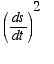(1) N (1) = 14(1)=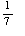,

it follows that

14(1)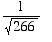=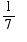and hence that(1) =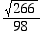=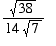.

As you can check, that agrees with the result from Formula (10) in Section 14.3.